﻿ 并列双圆柱流致振动的不对称振动和对称性迟滞研究
«上一篇文章快速检索 高级检索

 力学学报2015, Vol. 47Issue (5): 731-739  DOI: 10.6052/0459-1879-15-0070

### 引用本文 [复制中英文]

[复制中文]
Chen Weilin, Ji Chunning, Xu Wanhai. NUMERICAL INVESTIGATION ON THE ASYMMETRIC VIBRATION AND SYMMETRY HYSTERESIS OF FLOW-INDUCED VIBRATION OF TWO SIDE-BY-SIDE CYLINDERS[J]. Chinese Journal of Ship Research, 2015, 47(5): 731-739. DOI: 10.6052/0459-1879-15-007.
[复制英文]

### 文章历史

2015-01-06收稿
2015-06-06录用
2015-06-08网络版发表

Bearman和Wadcock的并列双圆柱绕流实验研究发现,在偏斜间隙流的影响下,并列双圆柱后的尾流呈现为双稳态,间隙流周期性地从一个方向变化到另一个方向. Kim和Durbin将该现象称之为flip-flopping (简称为FF),并进一步研究发现这种间隙流方向偏转来回切换的时间间隔要比旋涡脱落周期大几个量级,且随雷诺数的减小而增加[4, 6, 7, 8, 10]. FF现象中,宽尾流圆柱的脱涡频率和阻力较低,而窄尾流圆柱的脱涡频率和阻力则要大一些,这是因为窄尾流的剪切层卷起时形成的负压区更靠近圆柱的后侧. Williamson对雷诺数200的并列双圆柱绕流实验发现,一定条件下,间隙流始终偏向一个圆柱,而不发生周期性摆动,称为偏斜间隙流 (biased gap flow).

1 数值方法 1.1 控制方程

 $\frac{{\partial u}}{{\partial t}} = - \left( {u \cdot \nabla } \right)u - \nabla p + \nu {\nabla ^2}u + f$ (1)
 $\nabla \cdot u = 0$ (2)

 $\begin{array}{*{20}{l}} {{u^{n + 1}} = {u^n} + \delta t(\frac{3}{2}{h^n} - \frac{1}{2}{h^{n - 1}} - \frac{3}{2}\nabla {p^n} + \frac{1}{2}\nabla {p^{n - 1}}) + }\\ {\;\;\;\;\;\;\;\;\;{f^{n + \frac{1}{2}}}\delta t} \end{array}$ (3)
 $\nabla \cdot {u^{n + 1}} = 0$ (4)

 ${f^{n + 1/2}}\delta t = D\{ {V^{n + 1}} - I[{u^n} + \delta t{\mkern 1mu} (\frac{3}{2}{h^n} - \frac{1}{2}{h^{n - 1}} - \qquad \\ {\kern 1pt} {\kern 1pt} {\kern 1pt} {\kern 1pt} {\kern 1pt} {\kern 1pt} {\kern 1pt} {\kern 1pt} {\kern 1pt} {\kern 1pt} {\kern 1pt} {\kern 1pt} {\kern 1pt} {\kern 1pt} {\kern 1pt} {\kern 1pt} {\kern 1pt} {\kern 1pt} {\kern 1pt} {\kern 1pt} {\kern 1pt} {\kern 1pt} {\kern 1pt} {\kern 1pt} {\kern 1pt} {\kern 1pt} {\kern 1pt} {\kern 1pt} {\kern 1pt} {\kern 1pt} {\kern 1pt} \frac{3}{2}\nabla {p^n} + \frac{1}{2}\nabla {p^{n - 1}})]\}$ (5)

 $m\frac{{{d^2}y}}{{d{t^2}}} + c\frac{{dy}}{{dt}} + ky = {F_y}$ (6)

1.2 问题描述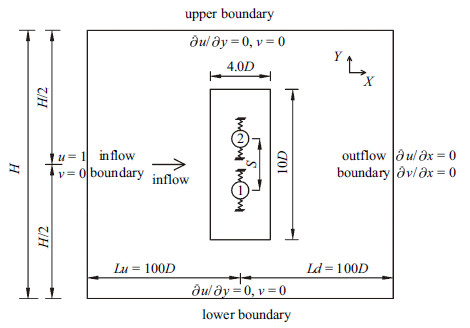图 1 计算域和边界条件 Fig.1 Computational domain and the boundary conditions

2 程序验证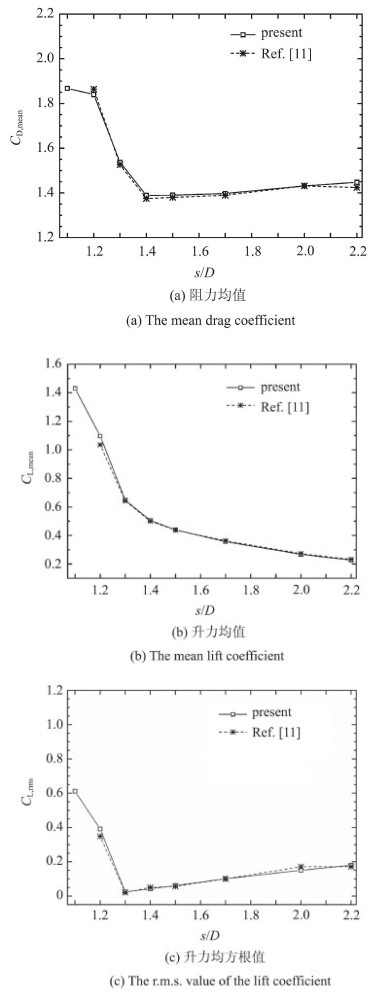图 2 本文结果与已有文献结果的对比 Fig.2 Comparison of present results with the published data
3 结果和讨论 3.1 并列双圆柱流致振动响应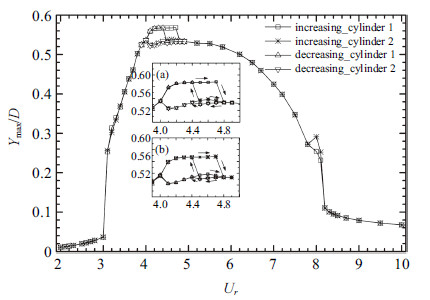图 3 并列双圆柱振幅随折合流速$U_r$的变化 ($s/D=2.5$) Fig.3 The vibration amplitude of two side-by-side circular cylinders versus $U_r$ at $s/D=2.5$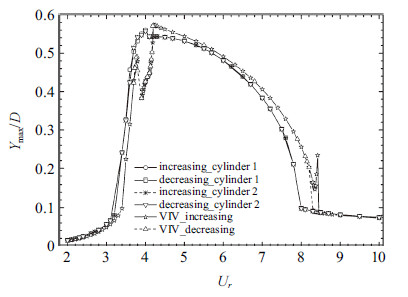图 4 并列双圆柱振幅随折合流速$U_r$的变化 ($s/D=5.0$) Fig.4 The vibration amplitude of two side-by-side circular cylinders versus $U_r$ at $s/D=5.0$
3.2 并列双圆柱流体力特性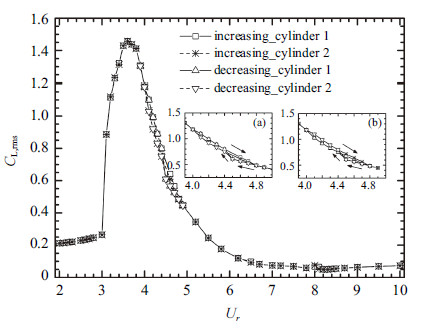图 5 升力均方根随折合流速变化 ($s/D=2.5$) Fig.5 The r.m.s. value of the lift coefficient versus $U_r$ at $s/D=2.5$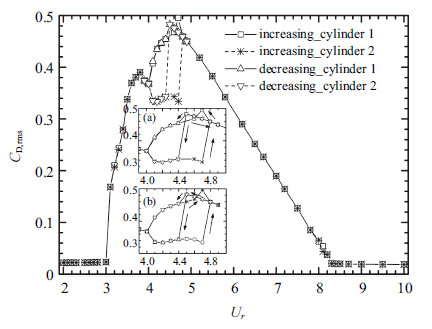图 6 阻力均方根随折合流速变化 ($s/D=2.5$) Fig.6 The r.m.s. value of the drag coefficient versus $U_r$ at $s/D=2.5$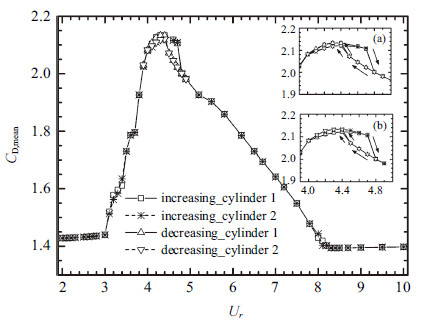图 7 阻力均值随折合流速变化 ($s/D=2.5$) Fig.7 The mean drag coefficient versus $U_r$ at $s/D=2.5$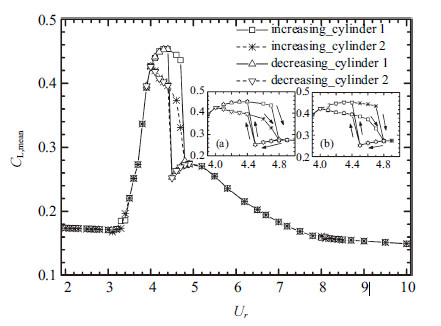图 8 升力均值随折合流速变化 ($s/D=2.5$) Fig.8 The mean lift coefficient versus $U_r$ at $s/D=2.5$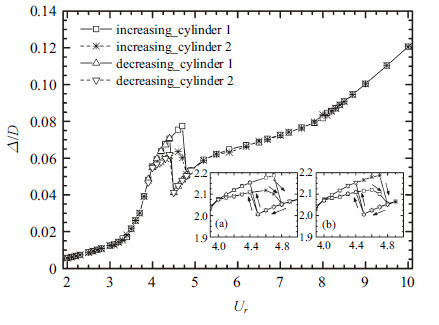图 9 平衡位置无量纲偏移量随折合流速变化 ($s/D=2.5$) Fig.9 The dimensionless shift of the balanced position versus $U_r$ at $s/D=2.5$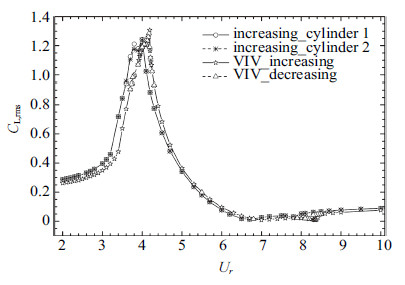图 10 升力均方根随折合流速变化 ($s / D = 5.0$) Fig.10 The r.m.s. value of the lift coefficient versus $U_r$ at $s / D = 5.0$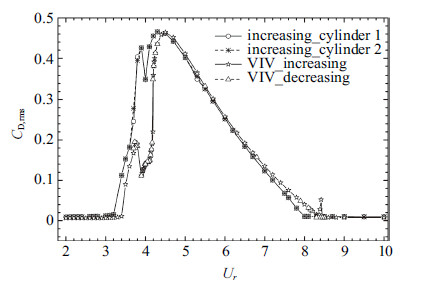图 11 阻力均方根随折合流速变化 ($s / D = 5.0$) Fig.11 The r.m.s. value of the drag coefficient versus $U_r$ at $s / D = 5.0$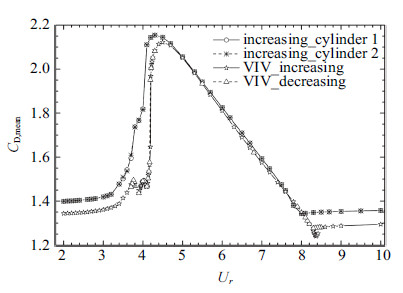图 12 阻力均值随折合流速变化 ($s / D = 5.0$) Fig.12 The mean drag coefficient versus $U_r$ at $s / D = 5.0$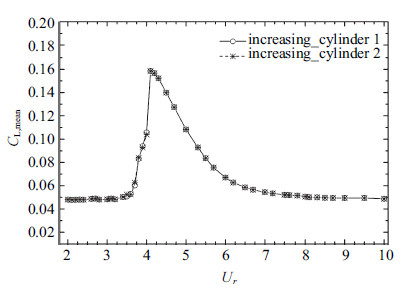图 13 升力均值随折合流速变化 ($s / D = 5.0$) Fig.13 The mean lift coefficient versus $U_r$ at $s / D = 5.0$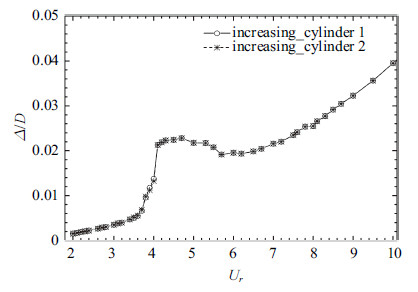图 14 平衡位置无量纲偏移量随折合流速变化 ($s / D = 5.0$) Fig.14 The dimensionless shift of the balanced position versus $U_r$ at $s / D = 5.0$

3.3 不对称振动和对称性迟滞现象的产生机制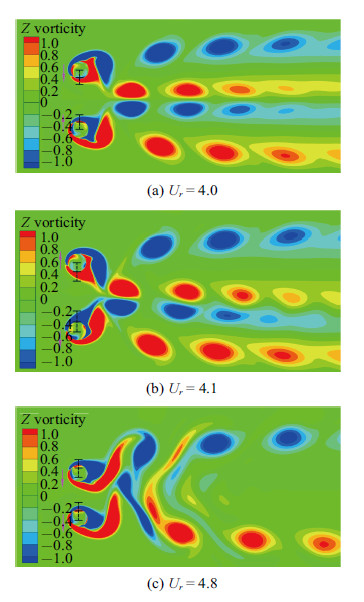图 15 不同折合流速下并列双圆柱流致振动的尾流模式 ($s/D = 2.5$) Fig.15 Wake patterns of flow-induced vibration of two side-by-side circular cylinders with different reduced velocities at the spacing ratio $s/D =2.5$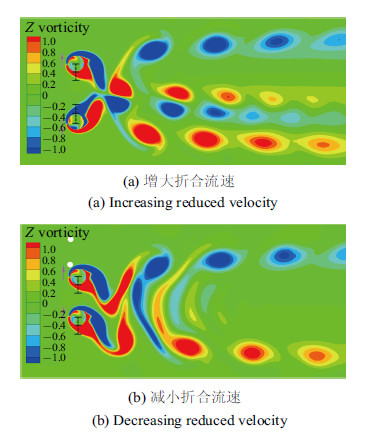图 16 $U_r = 4.6$时增大和减小折合流速并列双圆柱流致振动的尾流模式 ($s/D = 2.5$) Fig.16 Wake patterns of flow-induced vibration of two side-by-side circular cylinders with increasing and decreasing reduced velocities at $U_r = 4.6$ and $s/D = 2.5$
4 结 论

(1) 间距比$s/D=2.5$时,并列双圆柱流致振动中出现了不对称振动和对称性迟滞现象. 其中,不对称振动的折合流 速区间为$4.0 ＜U_r＜ 4.8$,对称性迟滞对应的折合流速区间为$4.4＜U_r ＜4.8$.

(2) 与振动响应相似,升力和阻力参数也在相同的折合流速区间内出现了不对称和迟滞现象.

(3) 通过对圆柱受力和尾流模式进行分析,解释了并列双圆柱流致振动中的不对称振动和对称性迟滞现象的产生机制. 两圆柱脱涡不完 全反相,其中一个圆柱的脱涡要早于另一个圆柱,造成间隙流稳定地偏斜,形成宽/窄尾流模式; 窄尾流圆柱的脱涡间距短,脱涡位置更靠近圆柱,圆柱受到的升阻力较大,造成圆柱的振幅较大; 相反,宽尾流圆柱的脱涡间距长,脱涡位置距离圆柱更远,圆柱受到的升阻力较小,造成圆柱的振幅较小. 圆柱的尾流模式具有一定的惯性'',倾向于维持原有的状态,这是形成对称性迟滞现象的原因.

(3) 间距比$s/D=5.0$时,并列双圆柱流致振动响应和受力与单圆柱涡激振动的非常接近,不对称振动和对称性迟滞现象消失.

  Sumner D. Two circular cylinders in cross-flow: A review. Journal of Fluids and Structures, 2010, 26: 849-899  Sumner D, Wong SST, Price SJ, et al. Fluid behavior of side-by-side circular cylinders in steady cross-flow. Journal of Fluids and Structures, 1999, 13: 309-338  Alam MM, Zhou Y. Flow around two side-by-side closely spaced circular cylinders. Journal of Fluids and Structures, 2007, 23: 799-805  Bearman PW, Wadcock AJ. The interaction between a pair of circular cylinders normal to a stream. Journal of Fluid Mechanics, 1973, 61: 499-511  Williamson CHK. Evolution of a single wake behind a pair of bluff bodies. Journal of Fluid Mechanics, 1985, 159: 1-18  Kim HJ, Durbin PA. Investigation of the flow between a pair of circular cylinders in the flopping regime. Journal of Fluid Mechanics, 1988, 196: 431-448  Alam MM, Moriya M, Sakamoto H. Aerodynamic characteristics of two side-by-side circular cylinders and application of wavelet analysis on the switching phenomenon. Journal of Fluids and Structures, 2003, 18: 325-346  Zhou Y, Zhang HJ, Yiu MW. The turbulent wake of two side-by-side circular cylinders. Journal of Fluid Mechanics, 2002, 458: 303-332  Meneghini JR, Satara F, Siqueira CLR, et al. Numerical simulation of flow interference between two circular cylinders in tandem and side-by-side arrangements. Journal of Fluids and Structures, 2001, 15: 327-350  Chen L, Tu JY, Yeoh GH. Numerical simulation of turbulent wake flows behind two side-by-side cylinders. Journal of Fluids and Structures, 2003, 18: 387-403  Kang S. Characteristics of flow over two circular cylinders in a side-by-side arrangement at low Reynolds numbers. Physics of Fluids, 2003, 15: 2486-2498  Carini M, Giannetti F, Auteri F. On the origin of the flip-flop instability of two side-by-side cylinder wakes. Journal of Fluid Mechanics, 2014, 742: 552-576.  Xu YS, Liu Y, Xia Y, et al. Lattice-Boltzmann simulation of two-dimensional flow over two vibrating side-by-side circular cylinders. Physical Review E, 2008, 78: 046314  Bao Y, Zhou D, Tu JH. Flow characteristics of two in-phase oscillating cylinders in side-by-side arrangement. Computers & Fluids, 2013, 71: 124-145  Cui ZD, Zhao M, Teng B. Vortex-induced vibration of two elastically coupled cylinders in side-by-side arrangement. Journal of Fluids and Structures, 2014, 44: 270-291  Huera-Huarte FJ, Gharib M. Flow-induced vibrations of a side-by-side arrangement of two flexible circular cylinders. Journal of Fluids and Structures, 2011, 27: 354-366  Chen WL, Ji CN, Xu WH, et al. On the responses and wake patterns of two side-by-side elastically supported circular cylinders in uniform laminar flow. Journal of Fluids and Structure, 2015, 55: 218-236  及春宁, 刘爽, 杨立红等. 基于嵌入式迭代的高精度浸入边界法. 天津大学学报, 2014, 47(5): 377-382 (Ji Chunning, Liu Shuang, Yang Lihong, et al. An accurate immersed boundary method based on built-in iterations. Journal of Tianjin University, 2014, 47(5): 377-382 (in Chinese))  Sen S, Mittal S, Biswas G. Steady separated flow past a circular cylinder at low Reynolds numbers. Journal of Fluid Mechanics, 2009, 620: 89-119.  及春宁, 陈威霖, 黄继露等. 串列双圆柱流致振动的数值模拟及其耦合机制. 力学学报, 2014, 46 (6) : 862-870 (Ji Chunning, Chen Weilin, Huang Jilu, et al. Numerical investigation on flow-induced vibration of two cylinders in tandem arrangements and its coupling mechanisms. Chinese Journal of Theoretical and Applied Mechanics, 2014, 46(6): 862-870 (in Chinese))
NUMERICAL INVESTIGATION ON THE ASYMMETRIC VIBRATION AND SYMMETRY HYSTERESIS OF FLOW-INDUCED VIBRATION OF TWO SIDE-BY-SIDE CYLINDERS
Chen Weilin, Ji Chunning, Xu Wanhai
State Key Laboratory of Hydraulic Engineering Simulation and Safety, Tianjin University, Tianjin 300072, China
Fund: The project was supported by the Science Fund for Creative Research Groups of the National Natural Science Foundation of China (51321065),the National Natural Science Foundation of China (51579175, 51479135) the Open Fund of State Key Laboratory of Hydraulics and Mountain River Engineering, Sichuan University (SKHL1303).
Abstract: Flow-induced vibration of two side-by-side circular cylinders at Re= 100 is numerically investigated. The cylinders are constrained to oscillate in the cross-flow direction with a center-to-center spacing ratio s/D = 2.5 and 5.0. The mass ratio of the cylinders is 2.0 and the reduced velocity is Ur= 2.0 ～ 10.0. The results show that, for the case with s/D = 2.5, asymmetric vibrations of the two side-by-side cylinders is observed in 4.0 < Ur<4.8, while the symmetry hysteresis is observed in 4.4 < Ur<4.8. However, for the case with s/D = 5.0, both two phenomena disappear and the vibration responses of two side-by-side circular cylinders are close to those of an isolated circular cylinder. Moreover, it was found that the lift and drag coefficients of the two cylinders also showed asymmetric features in the asymmetric vibrations region. When the two cylinders oscillate with different amplitudes, the gap flow stably biases to one of the cylinders and thus leads to a wide-narrow near-wake pattern. The cylinder with the narrower near-wake has larger vibration amplitude, drag and lift forces than the other one with the wider near-wake. A detailed explanation on the mechanisms of the asymmetric vibration and symmetry hysteresis is presented from the point of view of near-wake patterns before and after the asymmetric vibration occurs.
Key words: flow-induced vibration    two side-by-side cylinders    asymmetric vibration    symmetry hysteresis